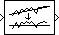# Detrend

Remove linear trend from vectors

•Libraries:
DSP System Toolbox / Statistics

## Description

The Detrend block removes a linear trend from the length-M input vector, u, by subtracting the straight line that best fits the data in the least squares sense.

The least squares line, û = ax + b, is the line with parameters a and b that minimizes the quantity

`$\sum _{i=1}^{M}{\left({u}_{i}-{\stackrel{^}{u}}_{i}\right)}^{2}$`

for M evenly-spaced values of x, where ui is the ith element in the input vector. The output, y = u-û, is always an M-by-1 column vector.

## Ports

### Input

expand all

Input data, specified as a vector. The block removes the straight line that best fits the data in the least squares sense.

Data Types: `single` | `double`

### Output

expand all

Output data, returned as a column vector. The block returns the remaining data after removing the straight line that best fits the data in the least squares sense.

Data Types: `single` | `double`

## Block Characteristics

 Data Types `double` | `single` Multidimensional Signals `No` Variable-Size Signals `No`

## Version History

Introduced before R2006a